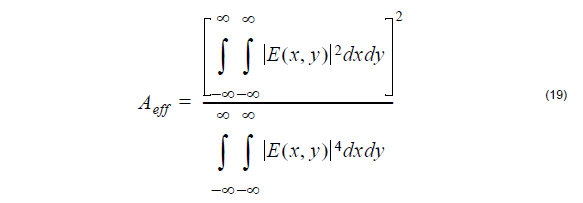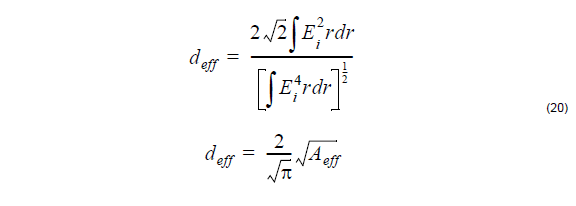## Mode field diameter and area importance

The Mode Field Diameter (MFD) is an important parameter related to the optical field
distribution in the fiber. It has been shown that MFD provides useful information about
the cabling performances, such as possible joint, macrobending, and microbending
losses. The effective area of the fibers has a direct relation to the nonlinear distortions
in long fiber links. Currently OptiFiber calculates the following spatial measures of the
modes:

## Near-field diameter definition

The near-field Mode Field Diameter (near-field MFD) is also known in the literature as
the “Petermann I” diameter. It is defined as the diameter at which the near field power
falls to 1 / eof its maximum value. It can be calculated by [Artiglia, 1989:where E(r) is the optical mode field distribution.

## Far-field diameter definition

The far-field Mode Field Diameter (far-field MFD) is also known in the literature as the
“Petermann II” diameter. It is defined as the diameter at which the far field power falls
to 1 / eof its maximum value. It can be calculated by [Artiglia, 1989]:where E(r) is the optical mode field distribution, and prime denotes differentiation of a
function with respect to its argument.

## Effective mode area definition

The effective Mode Area (eff. MA) is calculated as [see, for example, G. Agrawal,
1995]where E(x,y) is the optical mode field distribution.

## Effective diameter definition

OptiFiber calculates also the effective Mode Field Diameter (eff. MFD), defined as:where E(r) is the optical mode field distribution.